Next: Is there any efficient Up: Convex Polyhedron Previous: How can one remove   Contents

## Is there any efficient algorithm to remove redundant inequalities from a system of linear inequalities

This problem is essentially equivalent to the redundancy removal from point sets given in 2.20.

Although one can transform one to the other, let us describe a direct method. Let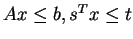be a given system of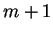-inequalities in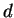-variables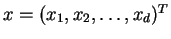. We want to test whether the subsystem of first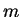inequalities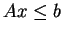implies the last inequality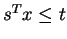. If so, the inequalityis redundant and can be removed from the system. A linear programming (LP) formulation of this checking is rather straightforward: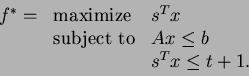(6)

Then the inequalityis redundant if and only if the optimal value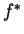is less than or equal to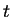.

By successively solving this LP for each untested inequality against the remaining, one would finally obtain a equivalent non-redundant system.

As we discussed in 2.20, one might be able to remove many redundant inequalities by using the same technique in dual form. Letbe the given system with high redundancy. The first step is to select a small subsystem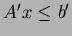of non-redundant inequalities from the original system. Typically such a system contains only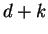inequalities for some small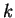(say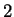or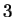). The second step is to compute all extreme points of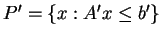. (Here we assume that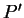is bounded, but one can generalize the technique for the unbounded case.) This is known as the vertex enumeration computation, 2.12. Clearlycontains the feasible region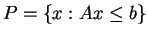. The final step is to test whether each original inequality is satisfied by all extreme points and rays. If so, the inequality is redundant for the subsystem and thus redundant for the original system.Next: Is there any efficient Up: Convex Polyhedron Previous: How can one remove   Contents
Komei Fukuda 2004-08-26